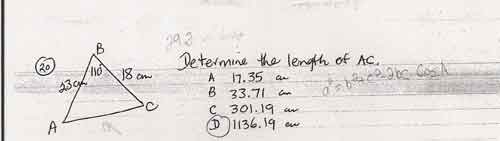SEARCH HOMEMath Central Quandaries & QueriesHey, my name is Candle I'm in academic math10 and am stuck on my trig... one question I thought I had right because i used the cosine law I got wrong and can't figure out why... here's a copy of the question. (i guessed it was D... but my teacher said it's B) Thanks CandleCandle sent us this imageCandle,

The cosine law says that b2 + c2 -bc cos(A) is equal to the square of a, not to a itself. So I'm guessing that when you saw answer D on your calculator you wrote it down and forgot that you still had a square root to take.

By the way, you should be able to do this question without a calculator! If you look at the problem, you see that two sides of the triangle are 18 and 23 cm long - that's small enough to draw on a standard sheet of paper at full size. Answers C [about 3m, roughly the height of a typical ceiling] and D [about 10m, higher than many houses] are obviously wrong. In particular, the "triangle inequality" says that each side of a triangle is less than the sum of the other two. So any answer bigger than 41cm can be ruled out immediately.

A cannot be right either because the given angle is bigger than 90 degrees, and a triangle can have only one such angle (the sum is 180 degrees). In a triangle, the bigger the angle the bigger the opposite side (the Sine Law says this quantitatively). Thus the missing side must be the longest side of the triangle, but the length given in A would be smallest.

Good Hunting!
RDMath Central is supported by the University of Regina and The Pacific Institute for the Mathematical Sciences.